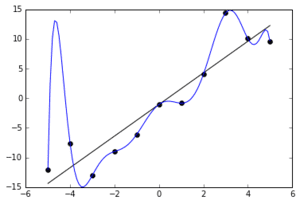# Brute force regression software?

Hi all

I have a lot of data, and was thinking if there exists a program that will apply a type of brute force regression tool to basically try any thinkable combination of variables and mathematical expressions to minimize the error between Y and Y_predicted.

The data [(x1 vs Y) (x2 vs Y)... (xn vs Y)] is very scattered data (random example below), so I will need something significantly more complicated than linear terms to get a nice Y to Y_predicted plot.

Br,
Peter

#### Attachments

•regression.jpg
39 KB · Views: 376

phyzguy
I don't think what you've asked for is really what you want. If you have N data points, an (N-1) dimensional polynomial will fit the data exactly, with zero error. However, such a model is probably physically meaningless. Usually you provide some physical insight of what the model should look like. What is your data, and what relationship do you expect between X and Y?

So you are saying that the fit depends only on the number of points in a variable, and not on the number of variables nor the variable dependencies - that sounds pretty strange.

Doesn't the N-1 rule apply to only a single variable with N values?

The purpose of the program would be to discover corrections between variables and start with simple relationships and try minimizing the error using various combinations. One would need some level of tolerance on the error, and the program would scale its complexity until an acceptable solution is found.

If there is enough data I guess that the correlation would eventually be meaningful, or what? One would obviously validate the model against some test data.

Is this in reality of problem for a neutral network? Problem with that is that I don't know if I have enough data..

Br, Peter

phyzguy
The point is that if I have some number of data points, I can always find a model where the number of free parameters in the model equals the number of parameters in the data. Then the model can fit the data exactly. We usually call this "overfitting" (see the example below). To do what you are proposing, you would have to quantitatively define the following:
(1) What makes a model "simple"? How do you measure the "complexity" a model? Which is more complex, a cubic polynomial, or an error function with one free parameter? Is it the number of free parameters? Or is a linear polynomial model simpler than some highly non-linear function.

I still think in order to do what you are trying to do, you need to inject some physical insight, and not search randomly through the infinite number of mathematical relationships between the variables.#### Attachments

•DrClaude
Baluncore
2021 Award
So, you have x1, x2 ... xn input variables.
For each value of y that you recorded, did you record what the values of all x1, x2 … xn were?

How many values of y did you record all the xi inputs?
Where is that data table?

Might be a good idea to try Fourier analysis, see if the findings hint at plausible processes, build hypotheses from such, test the resulting models...

I don't remember much from my Statistics courses, but one lecturer's dire warnings about 'Lies, Damned Lies and Inappropriate Correlations' still echo !!

Stephen Tashi
to basically try any thinkable combination of variables and mathematical expressions to minimize the error between Y and Y_predicted.

As others have pointed out, doing that literally would produce nonsensical results. However, there are more sophisticated approaches to fitting models that try to find a trade-off between the number of parameters in the model and the error in fit. This prevents getting an model that fits the data well but has a zillion parameters. The specific software to do this will depend on the general form of model you want. For example, look up info on ANOVA (Analysis of Variance) software.

Baluncore
2021 Award
How many values of y did you record all the xi inputs?
We still do not know if this is a Baysian or a least squares fitting problem.
There are software packages in the cloud that seek to find optimum equations and parameters for big data sets.

BvU
Homework Helper
I have a lot of data
Sure. Why not tell us how big your n is ?

The pictorial example looks pretty unreal.

If n is fairly small you might try the Tablecurve program

Else try PCA